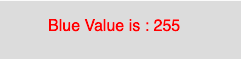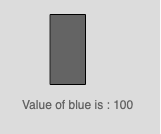# p5.js blue() function

The blue() function in p5.js is used to extract the blue value from a color or pixel array.

Syntax:

```blue(c)
```

Parameters: This function accepts single parameter c which stores the p5.Color object, color components, or CSS color.

Below programs illustrate the blue() function in p5.js:

Example 1: This example uses blue() function to extract the blue value from a color.

 `function` `setup() { ` `     `  `    ``// Create Canvas of size 300*80 ` `    ``createCanvas(300, 80); ` `} ` ` `  `function` `draw() { ` `     `  `    ``// Set the background color ` `    ``background(220); ` `     `  `    ``// Initialize the parameter ` `    ``let c = color(0, 126, 255, 102); ` `     `  `    ``// Extract the blue value ` `    ``let y = blue(c); ` `     `  `    ``// Set the font size ` `    ``textSize(16); ` `     `  `    ``// Set the font color ` `    ``fill(color(``'red'``)); ` `     `  `    ``// Display result ` `    ``text(``"Blue Value is : "` `+ y, 50, 30); ` `} `

Output:Example 2: This example uses blue() function to extract the blue value and fill it as grayscale integer value.

 `function` `setup() { ` ` `  `    ``// Create Canvas of size 300*80 ` `    ``createCanvas(160, 180); ` `} ` ` `  `function` `draw() { ` `     `  `    ``// Set background color     ` `    ``background(220); ` `     `  `    ``// Initialize the color ` `    ``let c = color(0, 126, 100, 34); ` `     `  `    ``// Use blue() function ` `    ``let value = blue(c); ` `     `  `    ``// Fill the color ` `    ``fill(value); ` `     `  `    ``// Create rectangle ` `    ``rect(50, 15, 35, 70); ` `     `  `    ``// Display result ` `    ``text(``"Value of blue is : "` `+ value, 22, 110); ` `} `

Output:Reference: https://p5js.org/reference/#/p5/blue

Whether you're preparing for your first job interview or aiming to upskill in this ever-evolving tech landscape, GeeksforGeeks Courses are your key to success. We provide top-quality content at affordable prices, all geared towards accelerating your growth in a time-bound manner. Join the millions we've already empowered, and we're here to do the same for you. Don't miss out - check it out now!